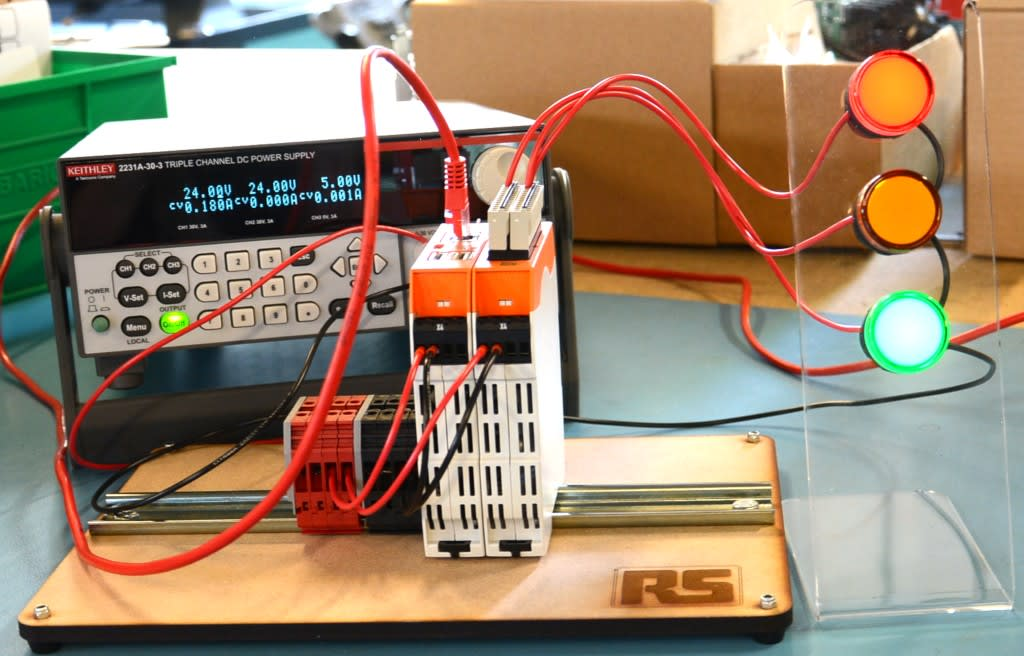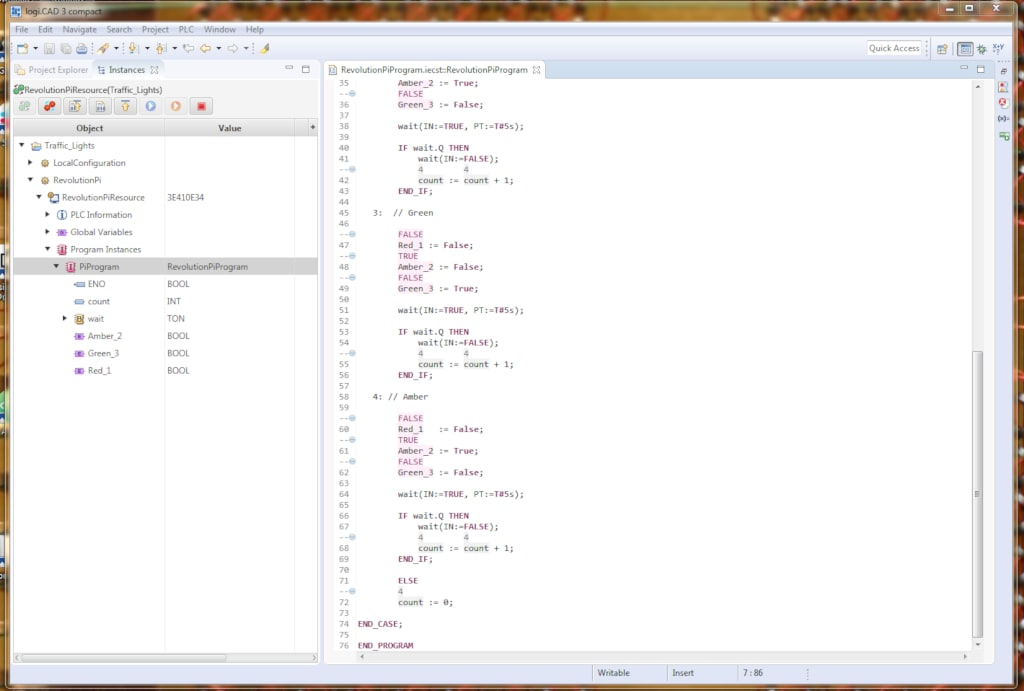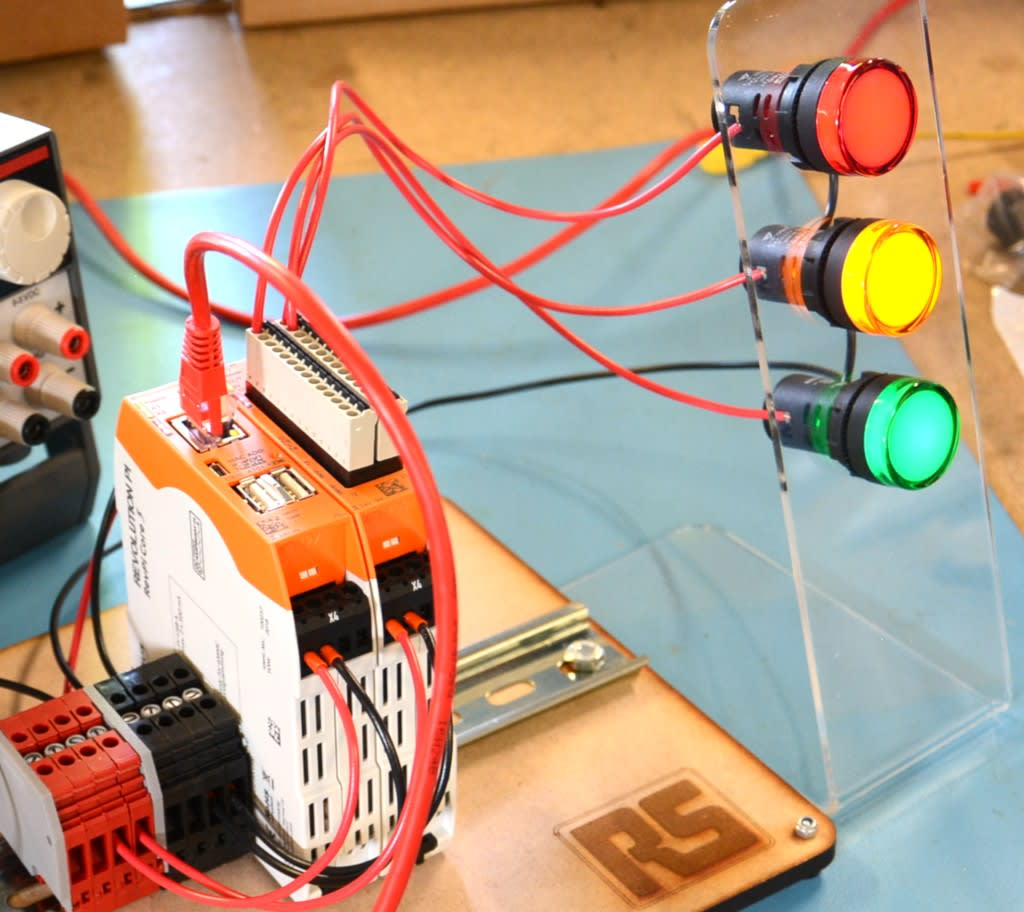# 通过结构式文本 (ST) 和 RevPi Core 3 控制交通灯### 结构式文本 (ST)• 对象 - logi.CAD 3 项目资源管理器中的对象位于文件夹内，是该文件夹的子文件夹。对象相当于文件系统中的文件。
• 项目 - 项目是 logi.CAD 3 项目资源管理器中的一种特殊类型的文件夹。默认情况下，项目是项目资源管理器中的根目录。

### 闪烁的灯光

``````PROGRAM RevolutionPiCounterProgram
VAR
value 	: BOOL;
END_VAR

VAR_EXTERNAL
Red_1 : BOOL;
Amber_2 : BOOL;
Green_3 : BOOL;
END_VAR

IF value THEN
Red_1 := False;
value := False;
ELSE
Red_1 := True;
value := True;
END_IF;

END_PROGRAM``````

### 交通信号灯TON 函数（计时器延迟）提供了所需的延迟，但我无法通过代码获得所需的效果。我能够使 LED 亮起一定时间后熄灭，但是却无法再次亮起，或者能够使 LED 闪烁并熄灭，但是却无法指定熄灭时间。我反复碰壁，一度觉得这项任务可能超出了我的处理能力。就在这时，我在网络上寻求帮助，在 Codesys 论坛上找到了一篇实用的博客文章，其中提到了 CASE 语句。Codesys 的功能与 logi.CAD 类似，而我发现它们所用的结构化文本语法存在一些差异，但是我成功地根据我的需要对示例进行了调整。

``````PROGRAM RevolutionPiProgram

VAR
wait : TON;
count : INT;
END_VAR

VAR_EXTERNAL
Red_1 : BOOL;
Amber_2 : BOOL;
Green_3 : BOOL;
END_VAR

CASE count OF
0: //init
count := count + 1;

1: // Red

Red_1 := True;
Amber_2 := False;
Green_3 := False;

wait(IN:=TRUE, PT:=T#5s);

IF wait.Q THEN
wait(IN:=FALSE);
count := count + 1;
END_IF;

2: // Red and Amber

Red_1 := True;
Amber_2 := True;
Green_3 := False;

wait(IN:=TRUE, PT:=T#5s);

IF wait.Q THEN
wait(IN:=FALSE);
count := count + 1;
END_IF;

3:  // Green

Red_1 := False;
Amber_2 := False;
Green_3 := True;

wait(IN:=TRUE, PT:=T#5s);

IF wait.Q THEN
wait(IN:=FALSE);
count := count + 1;
END_IF;

4: // Amber

Red_1 	:= False;
Amber_2 := True;
Green_3 := False;

wait(IN:=TRUE, PT:=T#5s);

IF wait.Q THEN
wait(IN:=FALSE);
count := count + 1;
END_IF;

ELSE
count := 0;

END_CASE;

END_PROGRAM``````

CASE 用于在出现特定整数值时执行语句。本例中创建了一个名为“count”的整数变量，此变量在每次 PLC 扫描时增加 1，直到最终条件运行以及 ELSE 将它重新设置为零。而 TON 将每个语句运行 5 秒。更改该数值时，只需编辑以下行中的 5s 值即可：

`wait(IN:=TRUE, PT:=T#5s);`

### 结论

I currently look after production at AB Open. I have a background in the arts, environmental conservation and IT support. In my spare time I do a bit of DJing and I like making things.